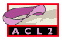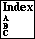UPDATE-NTH

modify a list by putting the given value at the given position
```Major Section:  PROGRAMMING
```

`(Update-nth key val l)` returns a list that is the same as the list `l`, except that the value at the `0`-based position `key` (a natural number) is `val`.

If `key` is an integer at least as large as the length of `l`, then `l` will be padded with the appropriate number of `nil` elements, as illustrated by the following example.

```ACL2 !>(update-nth 8 'z '(a b c d e))
(A B C D E NIL NIL NIL Z)
```
We have the following theorem.
```(implies (and (true-listp l)
(integerp key)
(<= 0 key))
(equal (length (update-nth key val l))
(if (< key (length l))
(length l)
(+ 1 key))))
```

The guard of `update-nth` requires that its first (position) argument is a natural number and its last (list) argument is a true list.